# Clustering basic benchmark

Cite as:
P. Fänti and S. Sieranoja
K-means properties on six clustering benchmark datasets
Applied Intelligence, 48 (12), 4743-4759, December 2018
https://doi.org/10.1007/s10489-018-1238-7
BibTex

Machine Learning
School of Computing
University of Eastern Finland
P.O.Box 111
FIN-80101 Joensuu
Finland

 S-sets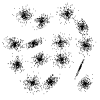S1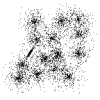S3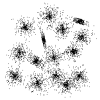S2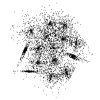S4 Synthetic 2-d data with N=5000 vectors and k=15 Gaussian clusters with different degree of cluster overlap P. Fränti and O. Virmajoki, "Iterative shrinking method for clustering problems", Pattern Recognition, 39 (5), 761-765, May 2006. (Bibtex) S1:  ts  txt S2:  ts  txt S3:  ts  txt S4:  ts  txt Ground truth centroids and partitions:  zip s3 and s4 updated 4.2.2015 A-setsA1 N=3000, k=20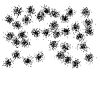A2 N=5250, k=35 Synthetic 2-d data with increasing number of clusters (k). There are 150 vectors per cluster. I. Kärkkäinen and P. Fränti, "Dynamic local search algorithm for the clustering problem", Research Report A-2002-6 (pdf)(Bibtex) A1:  ts  txt A2:  ts  txt A3:  ts  txtA3 N=7500, k=50 Ground truth centroids:   cb and txt Ground truth partitions:   pa Birch-sets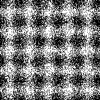Birch1Birch2 Synthetic 2-d data with N=100,000 vectors and k=100 clusters Zhang et al., "BIRCH: A new data clustering algorithm and its applications", Data Mining and Knowledge Discovery, 1 (2), 141-182, 1997. (Bibtex) Data sets (TS and TXT), ground truth centroids (CB and TXT) and partitions (PA):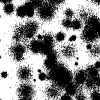Birch3 Birch1: Clusters in regular grid structure  ts  txt  cb  gt  pa  Birch2: Clusters at a sine curve  ts  txt cb  gt  pa  Birch3: Random sized clusters in random locations  ts  txt  cb  gt  Birch2 subsets: Varying N=1,000-1,000,000  ts  txt   Varying k=1-100  ts  txt G2 sets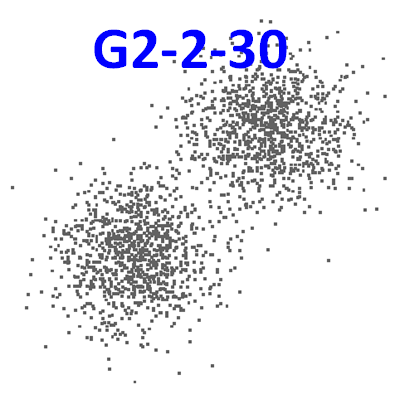G2 datasets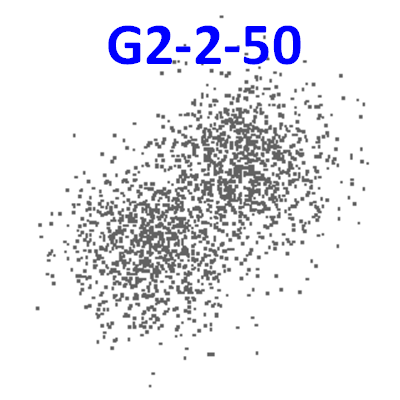N=2048, k=2 D=2-1024 var=10-100 Gaussian clusters datasets with varying cluster overlap (var) and dimensions (D). txt (17 MB)   ts (50 MB)   P. Fränti R. Mariescu-Istodor and C. Zhong, "XNN graph" IAPR Joint Int. Workshop on Structural, Syntactic, and Statistical Pattern Recognition Merida, Mexico, LNCS 10029, 207-217, November 2016. (Bibtex) Ground truth centroids: cb and txt Ground truth partitions: pa DIM-sets (high)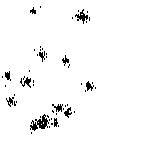dim032 D=32dim064 D=64 High-dimensional data sets N=1024 and k=16 Gaussian clusters. Clusters are well separated even in the higher dimensional cases. P. Fränti, O. Virmajoki and V. Hautamäki, "Fast agglomerative clustering using a k-nearest neighbor graph", IEEE Trans. on Pattern Analysis and Machine Intelligence, 28 (11), 1875-1881, November 2006. (Bibtex) Ground truth centroids: cb and txt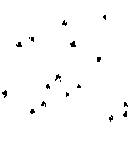dim128 D=128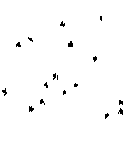dim256 D=256 Data sets in TS and TXT, ground truth partitions in PA format: dim032:  ts  txt  pa dim064:  ts  txt  pa dim128:  ts  txt  pa dim256:  ts  txt  pa dim512:  ts  txt  pa dim1024:  ts  txt  padim512 D=512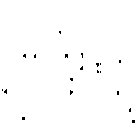dim1024 D=1024 DIM-sets (low)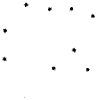Dim2 Synthetic data with Gaussian clusters. N=1351-10126 vectors in k=9 clusters in 2-15 dimensional space I. Kärkkäinen and P. Fränti, "Gradual model generator for single-pass clustering", Pattern Recognition, 40 (3), 784-795, March 2007. (Bibtex) ts  txt
 Unbalance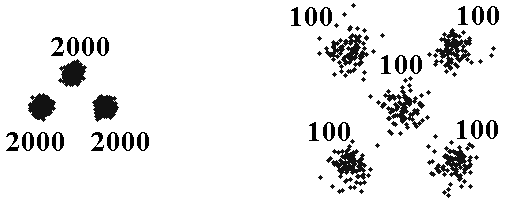Unbalance N=6500, k=8 Synthetic 2-d data with N=6500 vectors and k=8 Gaussian clusters ts  txt M. Rezaei and P. Fränti, "Set-matching measures for external cluster validity", IEEE Trans. on Knowledge and Data Engineering, 28 (8), 2173-2186, August 2016. (Bibtex) Ground truth centroids:   cb and txt Ground truth partitions:   pa

# Other clustering datasets

To cite the datasets please use the original articles.

 Image data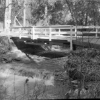Bridge (256x256)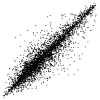N=4096, D=16 4x4 pixel blocks  ts  txt 4x4 binarized pixel blocks  ts  txt 4x4 pixel blocks: 25% randomly sampled (for training)  ts  txt 4x4 pixel blocks: 75% randomly sampled (for testing)  ts  txtHouse(256x256)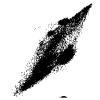N=34112, D=3 RGB-values, quantized to 5 bits per color  ts  txt RGB-values, 8 bits per color  ts  txtMiss America(360x288)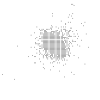N=6480, D=16 4x4 pixel blocks from the difference image of frame 1 and 2  ts  txt 4x4 pixel blocks from the difference image of frame 2 and 3  ts  txt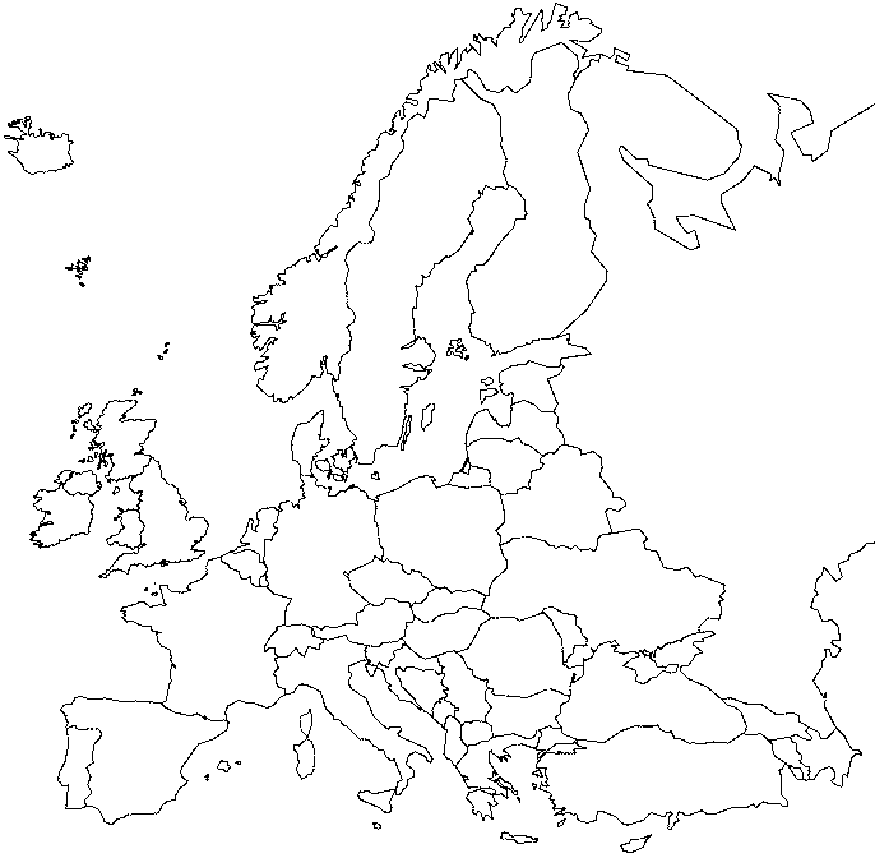Europe (vector)Europe N=169308, D=2 Differential coordinates of Europe map ts  txt  original  P. Fränti, M. Rezaei and Q. Zhao, "Centroid index: cluster level similarity measure", Pattern Recognition, 47 (9), 3034-3045, September 2014, 2014. (Bibtex) Nested datasets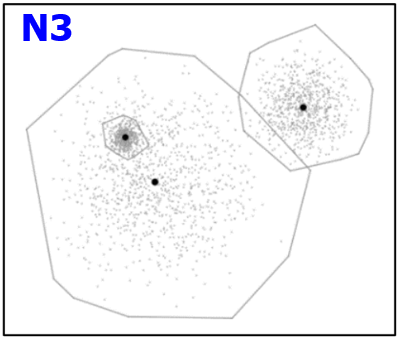N3 k=3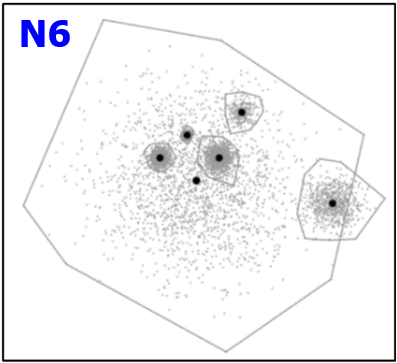N6 k=6 Nested Gaussian clusters N3 (N=2250) and N6 (N=5500). P. Fränti et al., "Article to be written". zip Worms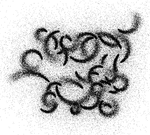Worms N=105,600, k=35, D=2 N=105,000, k=25, D=64 Synthetic 2-d and 64-d data with worm like shapes. Dataset and MATLAB generation scripts: worms.zip  S. Sieranoja and P. Fränti, "Fast and general density peaks clustering", Pattern Recognition Letters, 128, 551-558, December 2019. (pdf) Variations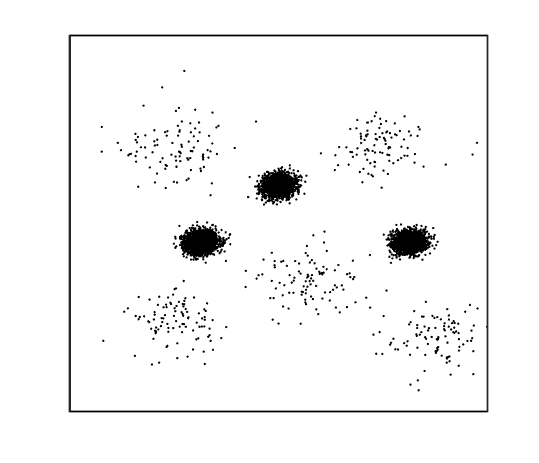Unbalance2 N=6500, k=8 ts  txt gt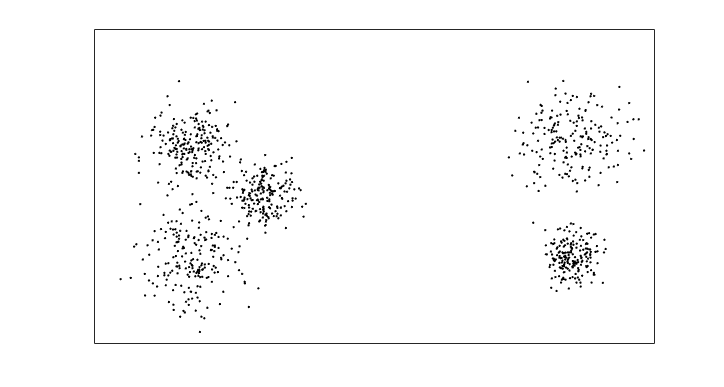Asymmetric N=1000, k=5 ts  txt gt Synthetic 2-d Gaussian clusters to test variations in cluster size unbalanace, symmetry, overlap and skewness M. Rezaei and P. Fränti, "Can the number of clusters be determined by external indices?", IEEE Access, 8 (1), 89239-89257, December 2020 (pdf).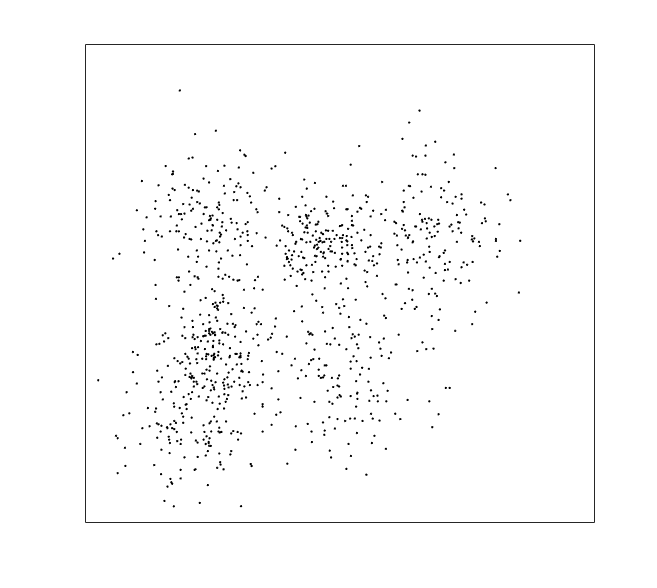Overlap N=1000, k=6 ts  txt gt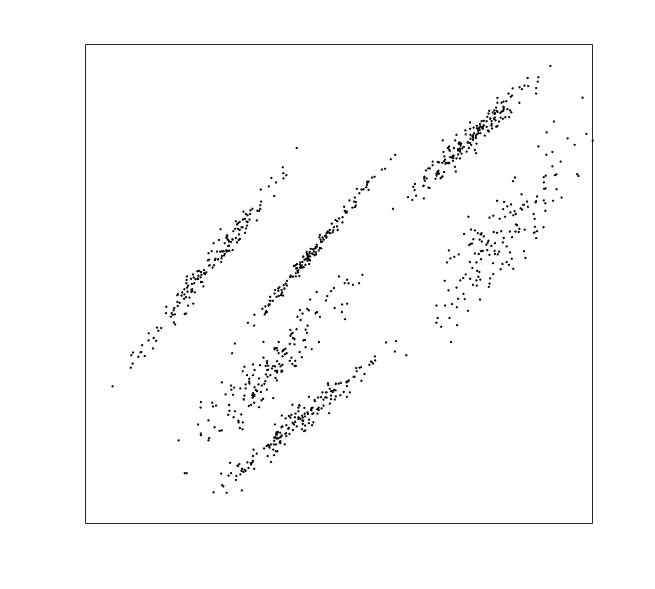Skewed N=1000, k=6 ts  txt gt
 Graph datasets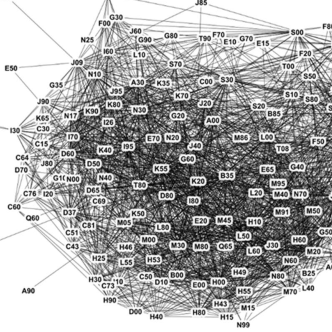varDeg: Artificial graphs, varying average degree varMu: Artificial graphs, varying mixing parameter mu (cluster overlap) varN: Artificial graphs, varying number of nodes icd10: Disease co-occurence networks Dataset: gclu_data.zip (437 MB) S. Sieranoja and P. Fränti, "Adapting k-means for graph clustering" Knowledge and Information Systems (KAIS), 4:1-28, December 2021. (pdf) More information here K-Sets dataSets data N=1200 k=4,8,16,32 D=100,200,400,800 Overlap=0,5%,10%,20%,40% Imbalance types=1,2,3,4,515 synthetic datasets of sets with N=1200 vectors and diverse number of clusters, dimensionality, overlap, and imbalance types Items of sets are codes for classification of diseases (ICD-10) introduced by World Health Organization (WHO). Data   Ground truth   Data generator M. Rezaei and P. Fränti, "K-sets and k-swaps algorithms for clustering sets", Pattern Recognition, 139, 109454, July 2023. (pdf)
 KDDCUP04Bio setKDDCUP04Bio N=145751, k=2000, D=74 KDDCUP04Bio biology dataset. KDDCUP04Bio:  ts  txt Shape sets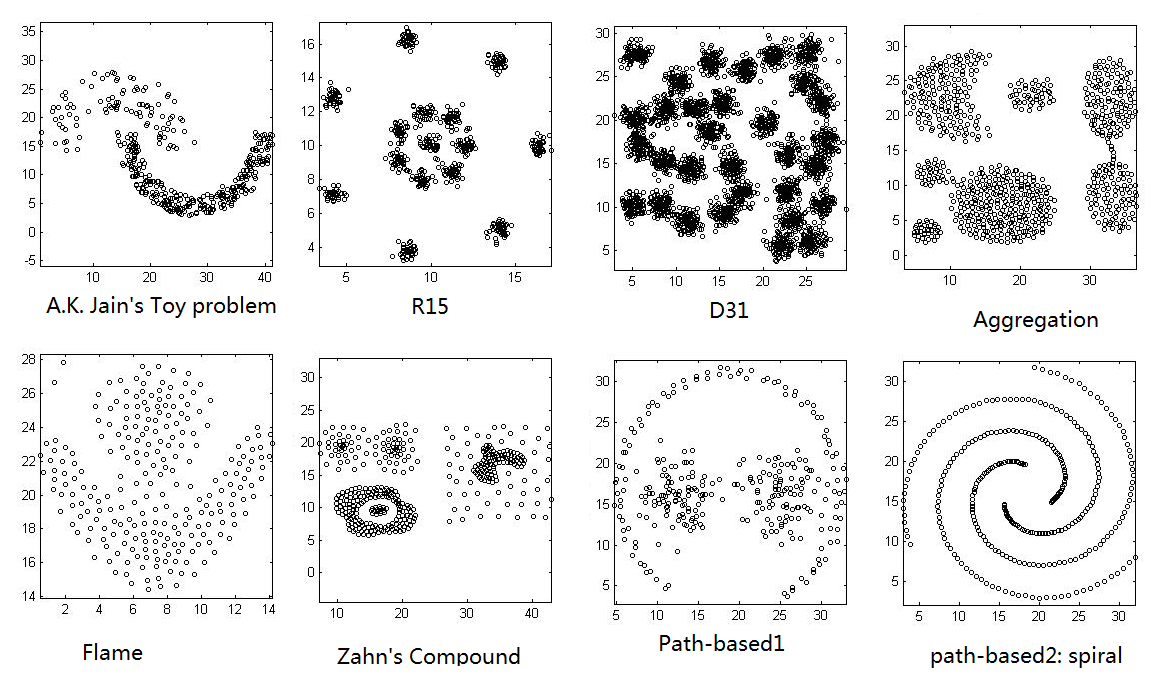Third column is the label. Aggregation N=788, k=7, D=2 Aggregation:  txt A. Gionis, H. Mannila, and P. Tsaparas, Clustering aggregation. ACM Transactions on Knowledge Discovery from Data (TKDD), 2007. 1(1): p. 1-30. Compound N=399, k=6, D=2 Compound:  txt C.T. Zahn, Graph-theoretical methods for detecting and describing gestalt clusters. IEEE Transactions on Computers, 1971. 100(1): p. 68-86. Pathbased N=300, k=3, D=2 Pathbased:  txt H. Chang and D.Y. Yeung, Robust path-based spectral clustering. Pattern Recognition, 2008. 41(1): p. 191-203. Spiral N=312, k=3, D=2 Spiral:  txt H. Chang and D.Y. Yeung, Robust path-based spectral clustering. Pattern Recognition, 2008. 41(1): p. 191-203. D31 N=3100, k=31, D=2 D31:  txt C.J. Veenman, M.J.T. Reinders, and E. Backer, A maximum variance cluster algorithm. IEEE Trans. Pattern Analysis and Machine Intelligence 2002. 24(9): p. 1273-1280. R15 N=600, k=15, D=2 R15:  txt C.J. Veenman, M.J.T. Reinders, and E. Backer, A maximum variance cluster algorithm. IEEE Trans. Pattern Analysis and Machine Intelligence, 2002. 24(9): p. 1273-1280. Jain N=373, k=2, D=2 Jain:  txt A. Jain and M. Law, Data clustering: A user's dilemma. Lecture Notes in Computer Science, 2005. 3776: p. 1-10. Flame N=240, k=2, D=2 Flame:  txt L. Fu and E. Medico, FLAME, a novel fuzzy clustering method for the analysis of DNA microarray data. BMC bioinformatics, 2007. 8(1): p. 3.
 UCI datasets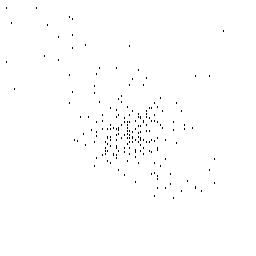Thyroid N=215, k=2, D=5 ts  txt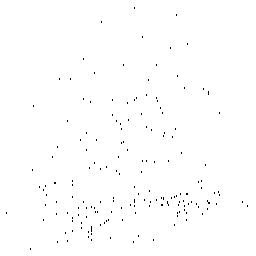Wine N=178, k=3, D=13 ts  txt UCI datasets original source is http://archive.ics.uci.edu/ml/ Breast-Cancer-Wisconsin: We have removed features 1 (sample id) and 11 (class label). All missing values are given value 1.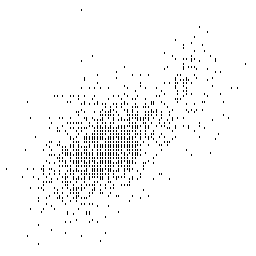Yeast N=1484, k=10, D=8 txt ts  integer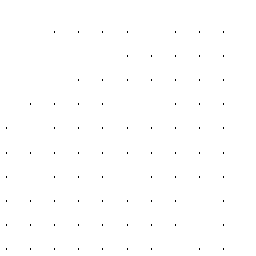Breast N=699, k=2, D=9 ts  txtIris N=150, C=3, D=4 ts  txt  labels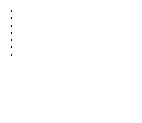Glass N=214, k=7, D=9, ts  txt  labels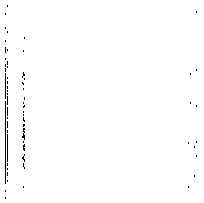Wdbc N=569, k=2, D=32 ts  full  numeric (D=31)leaves N=1600, k=100, D=64 zipLetter N=20000, k=26, D=16 zip
Categorical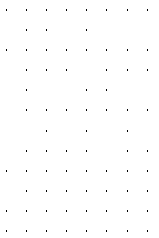Census
N=1000-512000, D=68
zip
Categorical attributes from Public Use Microdata Samples (PUMS) person records. Includes subsets of size 1000, 2000, 4000, ..., 512000. Source
Mopsi locationsUser locations
(Finland)
N=13467, D=2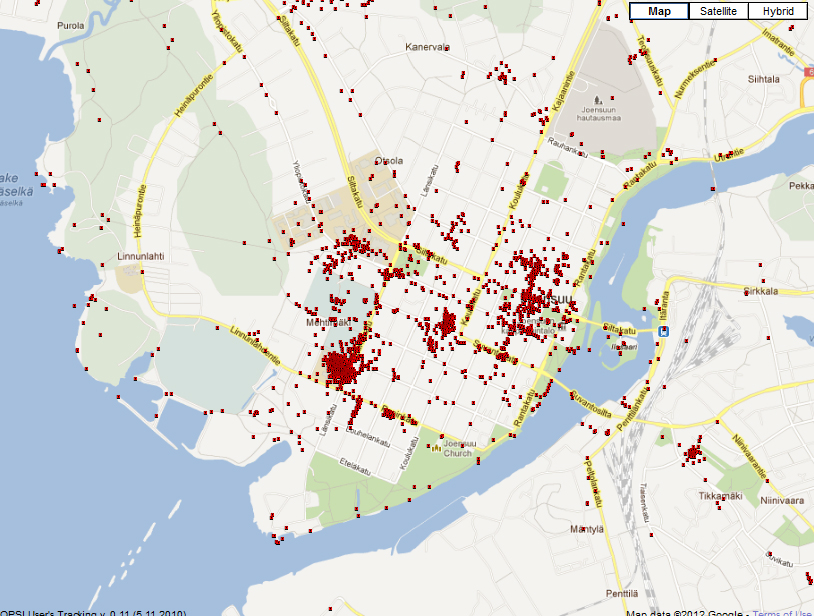User locations
(Joensuu)
N=6014, D=2

User locations until 2012 (FINLAND)
User locations:  cb  txt

User locations until 2012 (JOENSUU)
User locations Joensuu:  ts  txt

## Mopsi datasets

Miscellaneous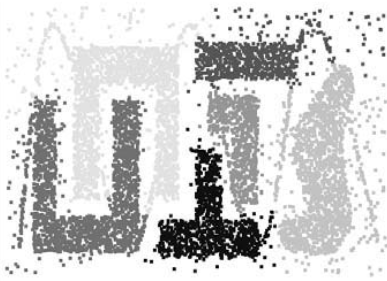t4.8k
N=8000, k=6, D=2
t4.8k.txtConfLongDemo
N=164,860, k=11, D=3
txt
t4.8k: G. Karypis, E.H. Han, V. Kumar, CHAMELEON: A hierarchical 765 clustering algorithm using dynamic modeling, IEEE Trans. on Computers, 32 (8), 68-75, 1999.

ConfLongdemo has eight attributes, of which only three numerical attributes are included here.MNIST
N=10000, k=10, D=748
txtMiniBooNE
N=130,065, D=50
txt
MNIST includes 10 handwriting digits and contains 60,000 477 training patterns and 10,000 test patterns of 784 dimensions.

MiniBooNE